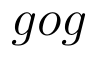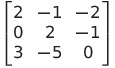Select Page

# CBSE Solutions for MCQ 12 Science Maths Differential Equations in Hindi

CBSE Solutions for MCQ 12 Science Maths Differential Equations in Hindi to enable students to get Solutions in a narrative video format for the specific question.

Expert Teacher provides CBSE Solutions for MCQ 12 Science Maths Differential Equations through Video Solutions in Hindi language. This video solution will be useful for students to understand how to write an answer in exam in order to score more marks. This teacher uses a narrative style for a question from Differential Equations not only to explain the proper method of answering question, but deriving right answer too.

Please find the question below and view the Solution in a narrative video format.

Question:

Solution Video in Hindi:

You can select video Solutions from other languages also. Please check Solutions in ( English )

## Similar Questions from CBSE, 12th Science, Maths, Differential Equations

Question 1 : Find the general solution of differential equation(View Answer Video)

Question 2 : Write the differential equation representing the family of curves y = mx, where m is an arbitrary constant. (View Answer Video)

Question 3 : Write the sum of the order and degree of the differential equation(View Answer Video)

Question 4 : Write the degree of the differential equation :(View Answer Video)

Question 5 : Find the solution of the differential equation(View Answer Video)

### Relations and Functions

Question 1 : Functionsare defined respectively, by, find(View Answer Video)

Question 2 : Number of binary operations on the set {a, b} are : (View Answer Video)

Question 3 : Let A = {1, 2, 3, 4} and let R = {(2, 2), (3, 3), (4, 4), (1, 2)} be a relation on A. Then, R is, (View Answer Video)

Question 4 :  * is a binary operation on Z such that: a * b = a + b + ab. The solution of (3* 4) *x = – 1 is,

Question 5 : Functionsare defined respectively, by, find(View Answer Video)

### Determinant

Question 1 : Find the adjoint  of the matrix. (View Answer Video)

Question 2 :  Evaluate the determinants:. (View Answer Video)

Question 3 : Evaluate the determinant:. (View Answer Video)

Question 4 : Let A be square matrix of order 3 * 3, then |kA|is equal to (View Answer Video)

Question 5 : Evaluate the determinant:. (View Answer Video)